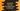# Find out the largest Date in a JavaScript array## Introduction :

Our problem is to find out the largest Date in a JavaScript array of dates. We will create one array of Date objects and then find out the largest among them.

I will show you different ways to solve this problem. Go through the examples and drop a comment below if you have any questions.

## Method 1: Using a loop :

Using a loop, we can iterate through the list elements and find out the largest one. In our case, the largest Date. We will use one extra variable to hold the largest value. Below is the program :

``````let dateArray = []

dateArray.push(new Date('2014/01/10'));
dateArray.push(new Date('2015/11/10'));
dateArray.push(new Date('2016/01/20'));
dateArray.push(new Date('2017/10/20'));

let maxDate = dateArray;
for (let date of dateArray) {
if (date > maxDate) {
maxDate = date;
}
}

console.log(`Max date = \${maxDate}`);
``````

Here, dateArray is an array of Date objects. maxDate is used to hold the largest Date object. At the start of the program, we are assigning the first element of dateArray to this variable. On each iteration of the loop, we are comparing it with the current value and if it is greater than maxDate, we are assigning it to maxDate.

maxDate will hold the largest object once the loop ends.

## Method 2: Using reduce :

reduce method can be used to find out the largest value in an array. We can pass one arrow function to reduce and based on this function, it will return the largest Date object.

``````let dateArray = []

dateArray.push(new Date('2014/01/10'));
dateArray.push(new Date('2015/11/10'));
dateArray.push(new Date('2016/01/20'));
dateArray.push(new Date('2017/10/20'));

let maxDate = dateArray.reduce((first,second) => first > second ? first : second);

console.log(`Max date = \${maxDate}`);
``````

Similarly, we can find out the smallest Date object.

## Method 3: Using sort :

sort is another way to find the largest Date in an array. We can sort the array in descending order and get the first element, which will be the largest.

``````let dateArray = []

dateArray.push(new Date('2014/01/10'));
dateArray.push(new Date('2016/11/10'));
dateArray.push(new Date('2018/01/20'));
dateArray.push(new Date('2015/10/20'));

let maxDate = dateArray.sort((first,second) => second - first);

console.log(`Max date = \${maxDate}`);
``````

## Method 4: Using Math.max.apply :

Math.max.apply can be used to find out the largest object in an array. It will return the largest value in milliseconds. So, you need to wrap it with Date constructor to get the Date object value.

``````let dateArray = []

dateArray.push(new Date('2014/01/10'));
dateArray.push(new Date('2016/11/10'));
dateArray.push(new Date('2018/01/20'));
dateArray.push(new Date('2015/10/20'));

let maxDate = new Date(Math.max.apply(undefined, dateArray));

console.log(`Max date = \${maxDate}`);
``````

Starting from ES6, you can use spread operator with Math.max like below :

``````let maxDate = new Date(Math.max(...dateArray));
``````